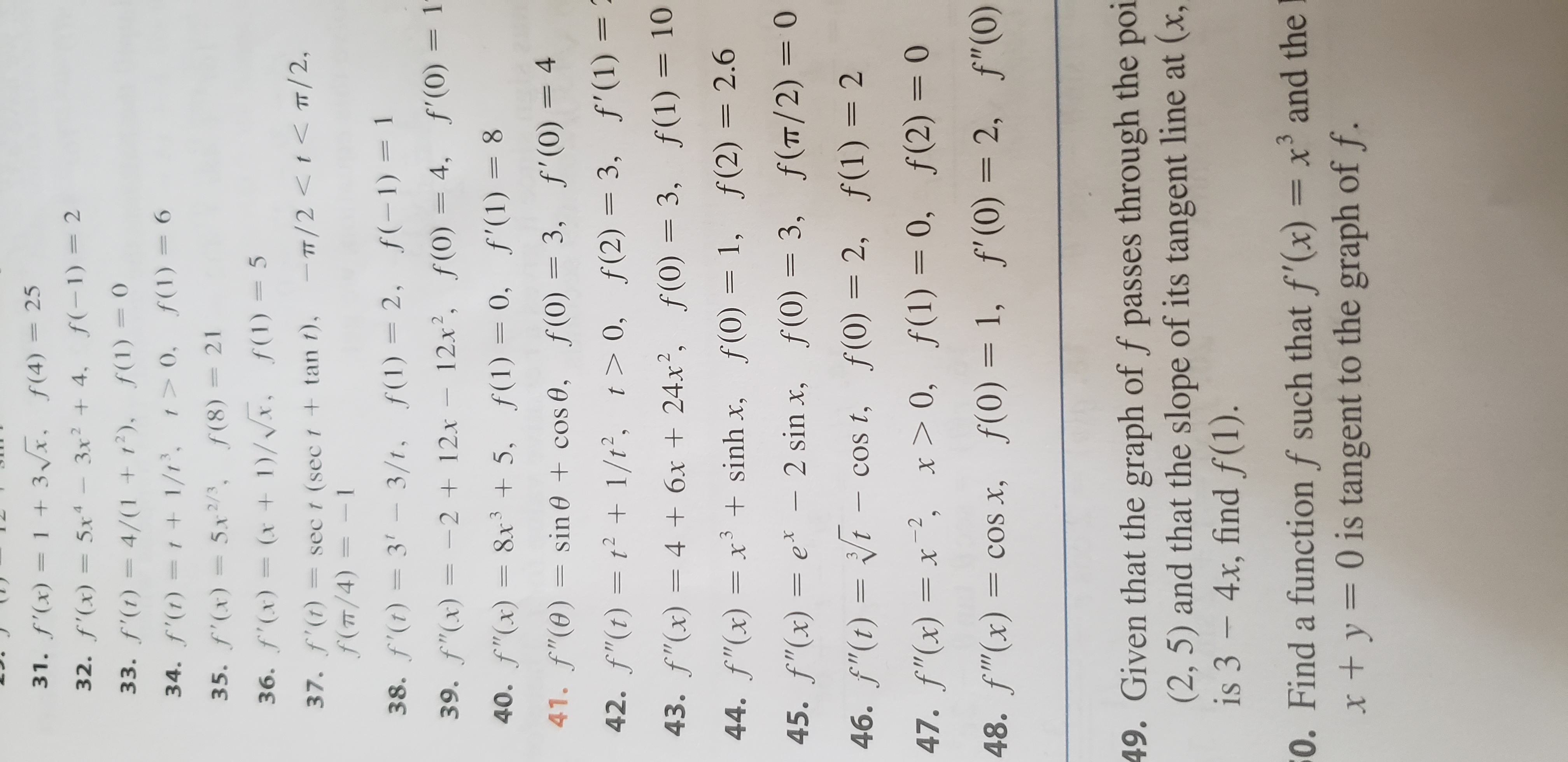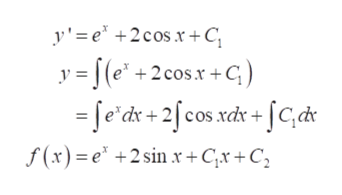# f'(x) = 1 +3x, f(4) 2532. f'(x) 5x3x4. f(1) 2f'(t) = 4/(1 + 12), f(1) 034. f'()=1 +1/1, 0, f(1)635. f'(x)5x2/, f(8)2136. f(x)=(x 1)/Vx, f1)= 5T/2 < 0,f(2) 3, f'(1) =43. f"(x)= 4 6x + 24x2, f(0)= 3, f(1)= 1044. f"(x)= x3 sinh x,f (0) = 1,f (2) 2.6ex- 2 sin x, f(0)= 3, f(T/ 2) = 045. f"(x)=t - cos t, f(0) = 2, f(1)= 246.f"(t)f(1) 23COs t,47. f"(x)= x-2, x> 0, f(1) = 0, f(2) = 048. f"(x)= coS x, f(0)= 1, f'(0)= 2, f"(0)49. Given that the graph of f passes through the poi(2, 5) and that the slope of its tangent line at (x,is 3 - 4x, find f(1).0. Find a function f such that f'(x)= x and thet y= 0 is tangent to the graph of f.11

Question
6 views

I need help with question #45 in Section 4.9, page 356, in the James Stewart Calculus Eighth Edition textbook. I need to find "f" given the function and the conditions.help_outlineImage Transcriptionclosef'(x) = 1 +3x, f(4) 25 32. f'(x) 5x 3x 4. f(1) 2 f'(t) = 4/(1 + 12), f(1) 0 34. f'()=1 +1/1, 0, f(1)6 35. f'(x)5x2/, f(8) 21 36. f(x)=(x 1)/Vx, f1)= 5 T/2 < 0, f(2) 3, f'(1) = 43. f"(x)= 4 6x + 24x2, f(0)= 3, f(1)= 10 44. f"(x)= x3 sinh x, f (0) = 1, f (2) 2.6 ex- 2 sin x, f(0)= 3, f(T/ 2) = 0 45. f"(x)= t - cos t, f(0) = 2, f(1)= 2 46.f"(t) f(1) 2 3 COs t, 47. f"(x)= x-2, x> 0, f(1) = 0, f(2) = 0 48. f"(x)= coS x, f(0)= 1, f'(0)= 2, f"(0) 49. Given that the graph of f passes through the poi (2, 5) and that the slope of its tangent line at (x, is 3 - 4x, find f(1). 0. Find a function f such that f'(x)= x and the t y= 0 is tangent to the graph of f. 11 fullscreen
check_circle

Step 1

Consider the given second order differential equation.

Step 2

First of all, integrate f ''(x) w.r.t x.help_outlineImage Transcriptionclosef"(x) =e* – 2sin .xr y"= e* – 2sin x y'= [(e* - 2 = fe'dx – 2[sin xdx sin x \d+ f '(x) = e* +2cos.x + C fullscreen
Step 3

Again, integrate f '(x) w.r...help_outlineImage Transcriptionclosey'e 2cosr+C y = [(e* +2cosx+G) =fe'dx+ 2[cos.xdx + fc,dc f(x)e +2sin x+ Cx +C fullscreen

### Want to see the full answer?

See Solution

#### Want to see this answer and more?

Solutions are written by subject experts who are available 24/7. Questions are typically answered within 1 hour.*

See Solution
*Response times may vary by subject and question.
Tagged in

### Differential Equations